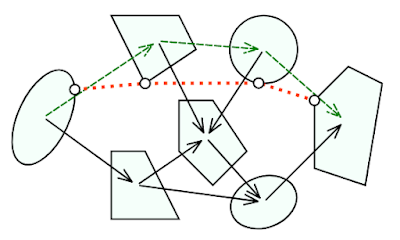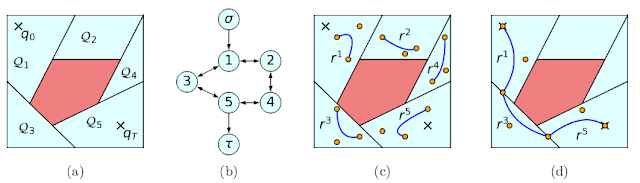### Differing Formulations of GCS for Motion Planning

In case you haven't heard of it before, GCS stands for "Graph of Convex Sets", and it is a framework for certain mixed integer convex optimization problems, primarily used for robot motion planning. I previously wrote a blog post on my experience implementing a basic version of it, which you can find here. I'd recommend reading that blog post before looking at this one.

If you've read that previous blog post, you may recall that I described two different ways of transcribing the motion planning problem into a graph of convex sets. I implemented one version, which seems more faithful to the original GCS paper. However, the other transcription, presented in the planning-specific followup paper, has several benefits over the previous approach. In this blog post, I'll briefly present both methodologies, and explain their costs and benefits.

## Formulation 1: Conjugate GraphFig. 1 of Shortest Paths in Graphs of Convex Sets

The classic picture of GCS is the one shown in Figure 1 of the original paper (included above). However, it's not immediately obvious how to use this for collision free motion planning. If the sets shown are regions which we know to be collision free, that says nothing about whether the paths between the sets are also collision free.

The idea behind the conjugate graph formulation is to use the intersections of collision-free sets as the sets in the graph. We can then move between two sets if the straight line path between them is contained within a collision-free set, i.e., if the intersections share a constituent set. In effect, we have interchanged the role of the sets and edges. The corresponding graph-theoretic construction is called the line graph, but it has many other names. I find that "line graph" is a rather ambiguous term, so I always refer to it as the conjugate graph.

## Formulation 2: Overlap GraphFig. 1 of Motion Planning around Obstacles with Convex Optimization

In generalizing the motion planning framework of GCS to handle Bézier curve trajectories, the authors use a different way of encoding the collision free sets. They place several points in each free region, and use them as control points to describe a segment of the trajectory. The trajectory endpoints are then constrained to be equal to each other on the overlap between each set (if the boolean variable corresponding to that edge being traversed is true).

In my experience with both formulations, I've found that the conjugate graph formulation is more straightforward to implement. But beyond that, there are few reasons to not go with the overlap graph formulation. The overlap graph formulation parameterizes a wider class of trajectories, enabling its use for broader optimization problems. It's also likely to be the "official" formulation going forward.

The conjugate graph does present a subtle benefit over the overlap graph, but it comes with a hidden cost. Suppose one wanted to preprocess the graph of convex sets, after a start and goal have been defined, but before formulating the optimization problem. Given a lower bound on each edge and vertex cost, and any feasible solution (which is easily obtainable via heuristic methods), it's possible to prune away many edges and sets if the cumulative best-case cost from the start or goal exceeds the incumbent solution. This could potentially dramatically reduce the size of the optimization problem.

In the conjugate graph formulation, it's easy to compute useful lower bounds. But in the overlap graph formulation, the lower bound is trivially zero, as all the points in the set could be made identical. However, there is no free lunch for the conjugate graph formulation. In scenarios where many of the convex sets overlap, the number of sets and edges in the conjugate graph can grow quite large. So the gains from the preprocessing may not outweigh the overall increase in problem size. Perhaps the conjugate graph lower bounds could be adapted to the overlap formulation, but I haven't come up with a way to to do this yet. GCS is still a young framework, and there is still a lot of room for clever heuristics, preprocessing techniques, and overall engineering effort to improve the algorithm.

Going back to the main point of this post, I think the overlap graph is the best formulation for actually using GCS in the future. (Even if it takes a little more effort to wrap your head around how it actually fits into the optimization framework.)